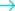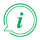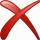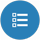# Convert density from Kilogram per cubic metre to Ounce per cubic inch## Convert from Kilogram per cubic metre

### About Kilogram per cubic metre to Ounce per cubic inch converter

##### Info

To convert from Kilogram per cubic metre to Ounce per cubic inch fill in the conversion tool field with the amount you want to convert. The result of Kilogram per cubic metre to Ounce per cubic inch conversion will be appeared in the "Result" field in red characters, without need of pressing any button. Below the conversion tool, a list will appear with all the available conversions from Kilogram per cubic metre.

#### Examples of Common Queries about converting Kilogram per cubic metre to Ounce per cubic inch

##### Kilogram per cubic metre to Ounce per cubic inch converter helps you to find a solution about:
• How do I turn Kilogram per cubic metre into Ounce per cubic inch?
• How to convert Kilogram per cubic metre to Ounce per cubic inch.
• How to make Kilogram per cubic metre Ounce per cubic inch.
• How do I convert Kilogram per cubic metre density to Ounce per cubic inch density ?
• Is Kilogram per cubic metre to Ounce per cubic inch converter free?
• Where can i find Kilogram per cubic metre to Ounce per cubic inch converter online.
• Is there a way to convert Kilogram per cubic metre to Ounce per cubic inch?